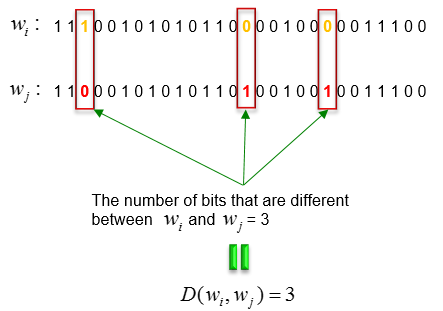Communication Technology Hamming Distance   Conceptually, Hamming Distance is a very simple concept. It indicates the number of bit differences between two binary data. I think just a couple of examples would be better than long lines of explanation.   For example, let's suppose that we are given two binary data A and B as shown below.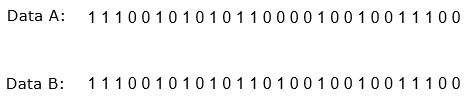Now you are asked to figure out how many bits are different between the data A and the data B. You can easily figure out the difference just comparing bit by bit. In this case, you would figure out only one bit difference as shown below.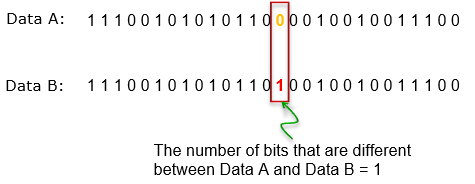Now let's figure out Hamming Distance between the two data. It is so simple. Just replace the term 'The number of bits that are different' with the term 'Hamming Distance' as shown below.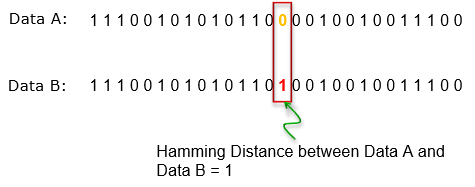In may text book or technical journals, they often use even more scary terms (mathematical expression) as shown below. This indicates Hamming Distance between codeword w(i) and w(j).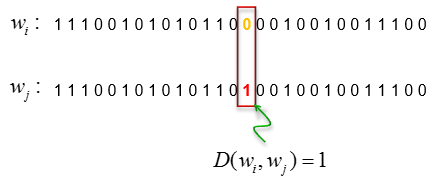As another example, you are given another data w(i) and w(j) as follows. The hamming distance can be calculated as follows.